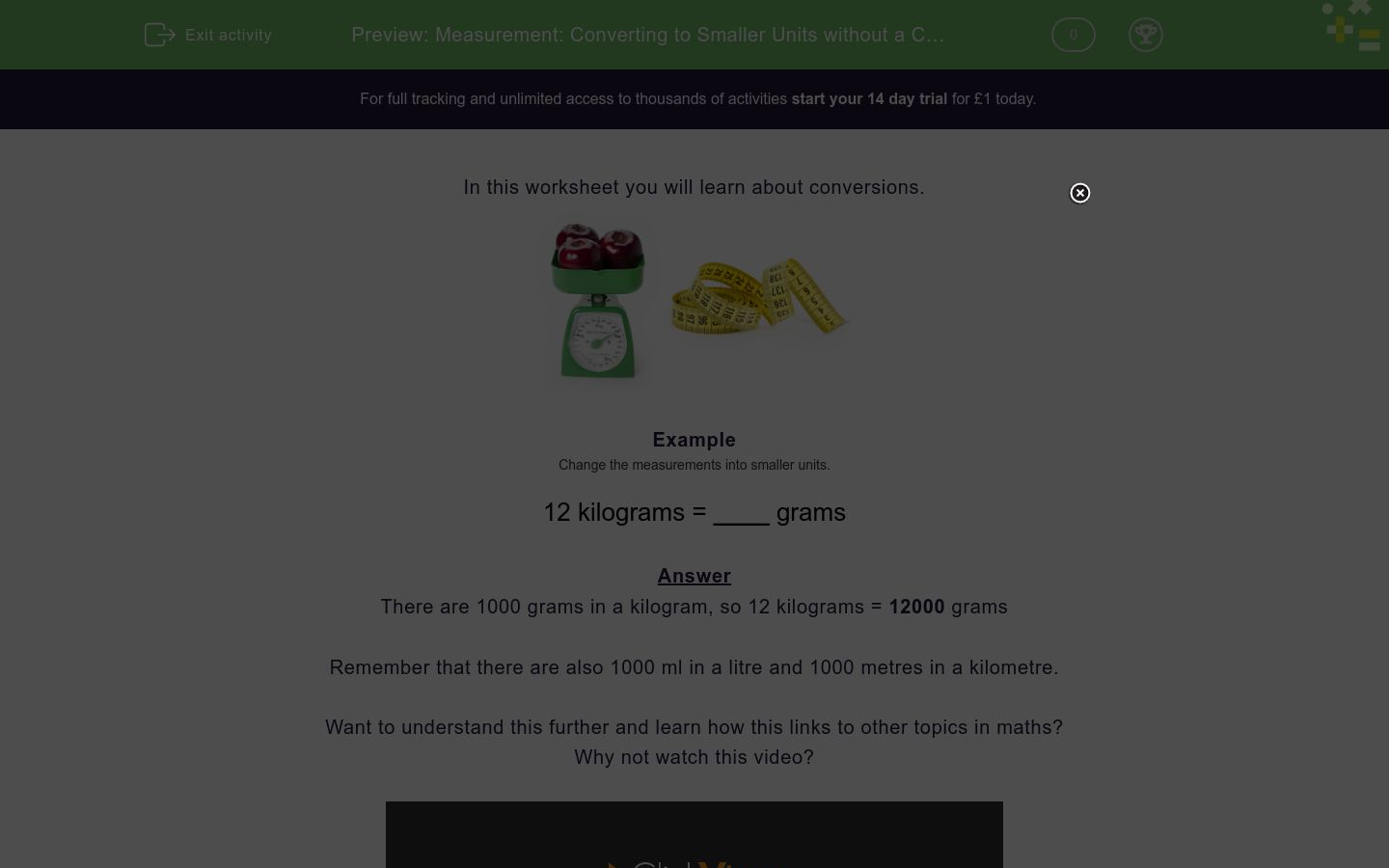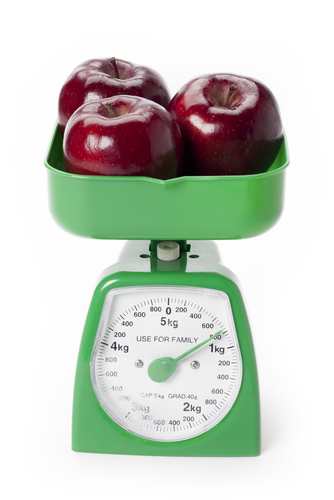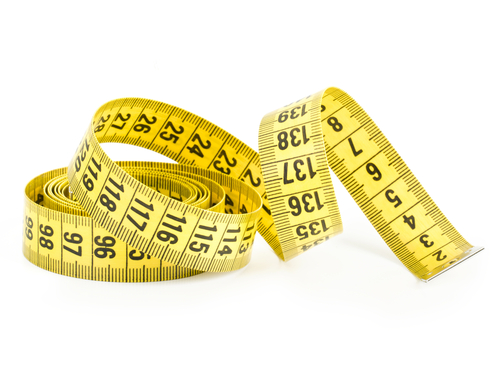# Measurement: Converting to Smaller Units without a Chart

In this worksheet, students convert measurements into smaller units by multiplying by 1000.Key stage:  KS 2

Curriculum topic:   Measurement

Curriculum subtopic:   Measure, Compare, Add/Subtract Lengths, Mass, and Volume/Capacity

Difficulty level:### QUESTION 1 of 10

In this worksheet you will learn about conversions.Example

Change the measurements into smaller units.

12 kilograms = ____ grams

There are 1000 grams in a kilogram, so 12 kilograms = 12000 grams

Remember that there are also 1000 ml in a litre and 1000 metres in a kilometre.

Want to understand this further and learn how this links to other topics in maths?
Why not watch this video?

Change the measurements into smaller units.

25 kilometres = _________ metres

Write the missing number below.

Change the measurements into smaller units.

44 kilograms = _________ grams

Write the missing number below.

Change the measurements into smaller units.

2 litres = _________ millilitres

Write the missing number below.

Change the measurements into smaller units.

64 kilometres = _________ metres

Write the missing number below.

Change the measurements into smaller units.

31 kilometres = _________ metres

Write the missing number below.

Change the measurements into smaller units.

2 kilograms = _________ grams

Write the missing number below.

Change the measurements into smaller units.

21 litres = _________ millilitres

Write the missing number below.

Change the measurements into smaller units.

16 kilograms = _________ grams

Write the missing number below.

Change the measurements into smaller units.

29 kilometres = _________ metres

Write the missing number below.

Change the measurements into smaller units.

32 kilograms = _________ grams

Write the missing number below.

• Question 1

Change the measurements into smaller units.

25 kilometres = _________ metres

Write the missing number below.

25000
EDDIE SAYS
25 × 1000
• Question 2

Change the measurements into smaller units.

44 kilograms = _________ grams

Write the missing number below.

44000
EDDIE SAYS
44 × 1000
• Question 3

Change the measurements into smaller units.

2 litres = _________ millilitres

Write the missing number below.

2000
EDDIE SAYS
2 × 1000
• Question 4

Change the measurements into smaller units.

64 kilometres = _________ metres

Write the missing number below.

64000
EDDIE SAYS
64 × 1000
• Question 5

Change the measurements into smaller units.

31 kilometres = _________ metres

Write the missing number below.

31000
EDDIE SAYS
31 × 1000
• Question 6

Change the measurements into smaller units.

2 kilograms = _________ grams

Write the missing number below.

2000
EDDIE SAYS
2 × 1000
• Question 7

Change the measurements into smaller units.

21 litres = _________ millilitres

Write the missing number below.

21000
EDDIE SAYS
21 × 1000
• Question 8

Change the measurements into smaller units.

16 kilograms = _________ grams

Write the missing number below.

16000
EDDIE SAYS
16 × 1000
• Question 9

Change the measurements into smaller units.

29 kilometres = _________ metres

Write the missing number below.

29000
EDDIE SAYS
29 × 1000
• Question 10

Change the measurements into smaller units.

32 kilograms = _________ grams

Write the missing number below.

32000
EDDIE SAYS
32 × 1000
---- OR ----

Sign up for a £1 trial so you can track and measure your child's progress on this activity.

### What is EdPlace?

We're your National Curriculum aligned online education content provider helping each child succeed in English, maths and science from year 1 to GCSE. With an EdPlace account you’ll be able to track and measure progress, helping each child achieve their best. We build confidence and attainment by personalising each child’s learning at a level that suits them.

Get started